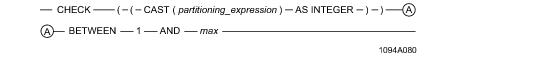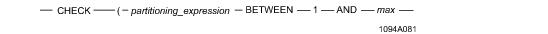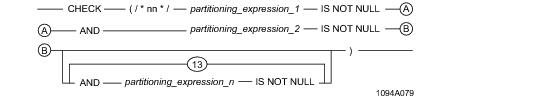# 15.00 - Partitioning CHECK Constraints for Partitioned Tables With 2-Byte Partitioning - Teradata Database

## Teradata Database SQL Data Definition Language Detailed Topics

Product
Release Number
15.00
Content Type
Programming Reference
Publication ID
B035-1184-015K
Language
English (United States)

### Partitioning CHECK Constraints for Partitioned Tables With 2‑Byte Partitioning

Teradata Database derives a table‑level partitioning CHECK constraint from the partitioning expression for partitioned tables. The text for this constraint, which is derived for table constraints, partitioning constraints, and named constraints cannot exceed 16,000 characters; otherwise, Teradata Database aborts the request and returns an error to the requestor.

The following diagrams provides the 2 forms of this partitioning CHECK constraint derived for single‑level partitioning. The forms differ depending on whether the partitioning expression has an INTEGER data type or not.

• The first form applies to single‑level 2‑byte partitioning expressions that do not have an INTEGER data type and are not defined only by a RANGE_N function.
• In this case, the type of the expression must be cast to INTEGER.• The second form applies to single‑level 2‑byte partitioning expressions that have an INTEGER data type.
•where:

 Syntax element … Specifies the … partitioning_expression partition number returned by the single‑level partitioning expression. max maximum number of partitions defined by partitioning_expression. If the partitioning expression is defined using something other than a RANGE_N or CASE_N function, the value of max is 65,535. If the partitioning expression is defined using only a RANGE_N or CASE_N function, the value of max is the number of partitions defined by the partitioning expression.

See “Rules and Usage Notes for Partitioned Tables” on page 625 and “Restrictions for Multilevel Partitioning” on page 634 for information about the table‑level partitioning CHECK constraints that Teradata Database creates for single‑level and multilevel partitioned primary index tables and join indexes.

• The third form applies to multilevel 2‑byte partitioning expressions.
• The following diagram provides the form of this partitioning CHECK constraint that Teradata Database derives for a multilevel partitioned primary index with 2‑byte partitioning.where:

 Syntax element … Specifies the … nn number of levels, or number of partitioning expressions, in the multilevel partitioning. nn can range between 02 and 15, inclusive. partitioning_expression_1 the partition number returned by the first multilevel partitioning expression. partitioning_expression_2 the partition number returned by the second multilevel partitioning expression. partitioning_expression_n the partition number returned by the nth multilevel partitioning expression. If the partitioning has 3 levels, there are 3 NOT NULL partitioning expressions in the implied constraint, if the partitioning has 10 levels, there are 10 NOT NULL partitioning expressions in the implied constraint, and so on.

For example, suppose you create the following table.

`     CREATE TABLE orders (`
`       o_orderkey      INTEGER NOT NULL,`
`       o_custkey       INTEGER,`
`       o_orderstatus   CHARACTER(1) CASESPECIFIC,`
`       o_totalprice    DECIMAL(13,2) NOT NULL,`
`       o_orderdate     DATE FORMAT 'yyyy-mm-dd' NOT NULL,`
`       o_orderpriority CHARACTER(21),`
`       o_clerk         CHARACTER(16),`
`       o_shippriority  INTEGER,`
`       o_comment       VARCHAR(79))`
`     PRIMARY INDEX (o_orderkey)`
`     PARTITION BY (RANGE_N(o_custkey BETWEEN 0`
`                                     AND 49999 `
`                                     EACH  100),`
`                   RANGE_N(o_orderdate BETWEEN DATE '2000-01-01'`
`                                       AND     DATE '2006-12-31'`
`                                       EACH INTERVAL '1' MONTH))`
`     UNIQUE INDEX (o_orderkey);`

The partitioning CHECK constraint SQL text that would be stored in DBC.TableConstraints for this multilevel partitioned primary index with 2‑byte partitioning is as follows.

`     CHECK (/*02*/ RANGE_N(o_custkey   BETWEEN 0 `
`                                       AND 49999 `
`                                       EACH  100) `
`                   IS NOT NULL`
`               AND RANGE_N(o_orderdate BETWEEN DATE '2000-01-01' `
`                                       AND     DATE '2006-12-31' `
`                                       EACH INTERVAL '1' MONTH) `
`                   IS NOT NULL )`

You could use the following SELECT request to retrieve index constraint information for each of the multilevel partitioned objects.

`     SELECT * `
`     FROM DBC.TableConstraints`
`     WHERE ConstraintType = 'Q' `
`     AND   SUBSTRING(TableCheck FROM 1 FOR 13) >= 'CHECK (/*02*/'`
`     AND   SUBSTRING(TableCheck FROM 1 FOR 13) <= 'CHECK (/*15*/';`